• Call Now

1800-102-2727•

# Plane mirrors, definition, ray diagrams, characteristics, uses, practice problems, FAQs

Let you be alone at your home. While playing you got injured on your forehead. Now you need first aid but as you cannot see your forehead, there will be difficulty in medicating. But if you use a plane mirror then you get a virtual image of your injury in the mirror and can medicate easily. Plane mirror is nothing but just a simple mirror at our homes. Lets see the working of the plane mirror!

Table of content

• What is a plane mirror?
• Object and image
• Ray diagram for the plane mirror
• Image formed by a plane mirror
• Characteristics of image formed by plane mirror
• Uses of plane mirror
• Practice problems
• FAQs

## What is a plane mirror?

A plane mirror is a reflecting surface made up of plane glass plate having a layer of reflecting material like silver nitrate or aluminum behind it. It works on the two laws of reflection.

The laws of reflection are-

1. The angle of incidence is equal to the angle of reflection (i = r).
2. The incident ray, the reflected ray, and the normal to the mirror at the point of incidence are in the same plane.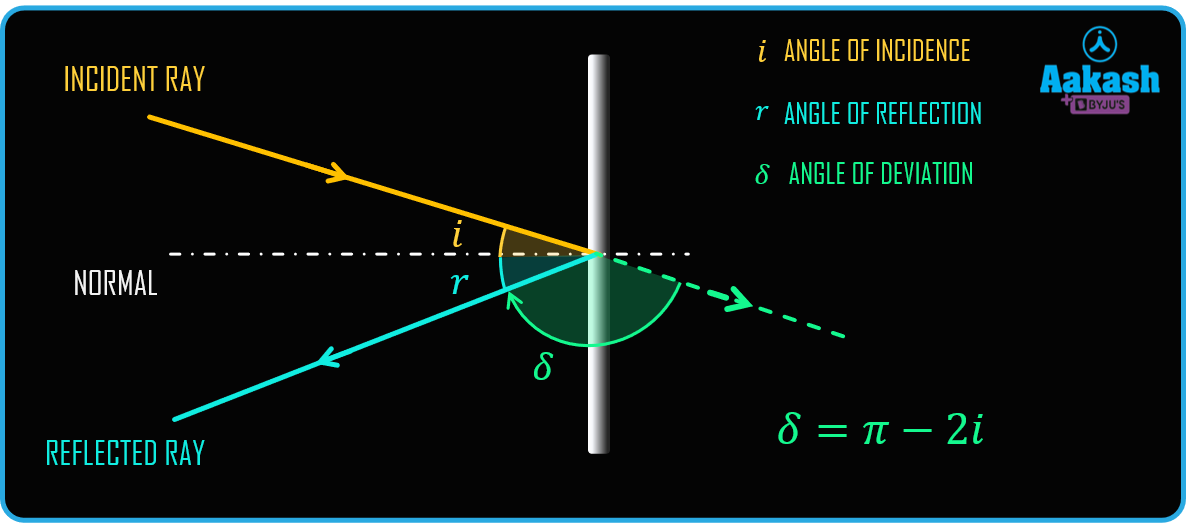From the above figure we can see a incident ray falls on the mirror at an angle of incident i, after the reflection it back at an angle r , then the angle of incident will be equal to angle of reflection. The angle of deviation is given as

$\delta =\mathbit{\pi }-2\mathbit{i}$

## Object and image

The intersection of incident rays is located as an object. If the incident rays intersect actually, then is it called an actual object and if the incident rays appear to meet then it is a virtual object.

The intersection of reflected rays is considered as an image. If the reflected rays actually meet then it is called an actual image and if the reflected rays appear to meet, then it is called a virtual image.

In the figure below we see the incident rays are actually meeting on O, so it is a real object and reflected rays appear to meet at I so it is a virtual image.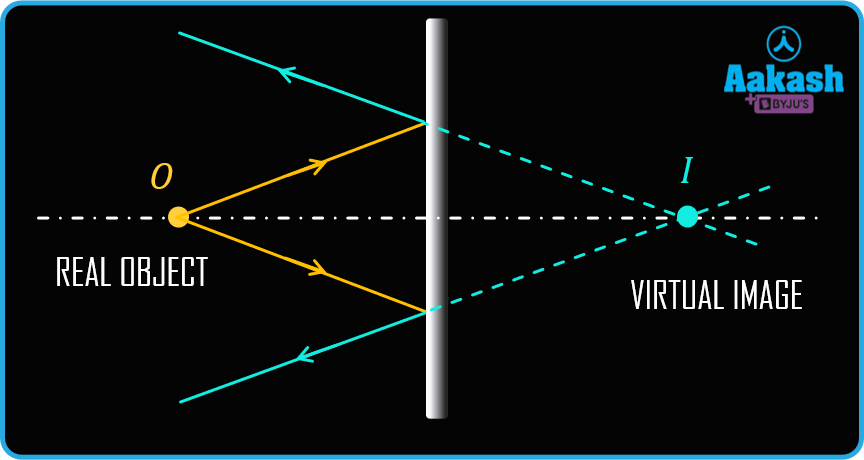Here in the figure below incident rays appear to meet at point O, so the object is virtual and after reflection the rays actually meet at I, so the image will be real.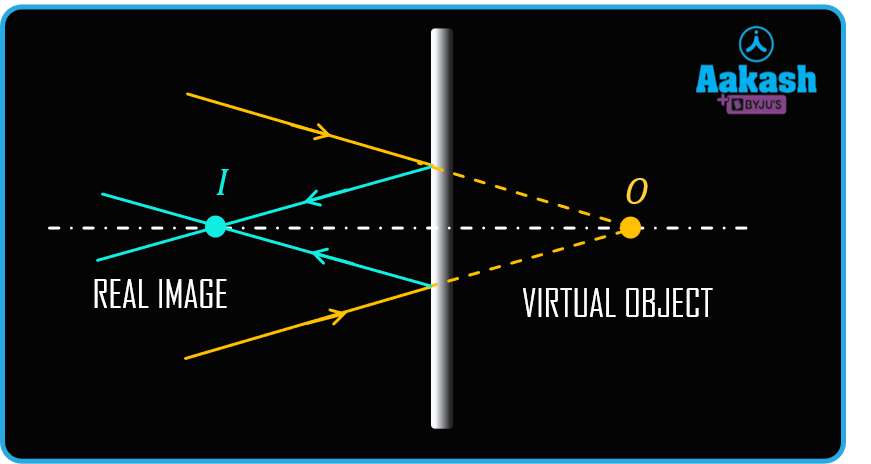## Ray diagram for the plane mirror

To understand the image formation through a plane mirror we have to understand the ray diagram of the plane mirror.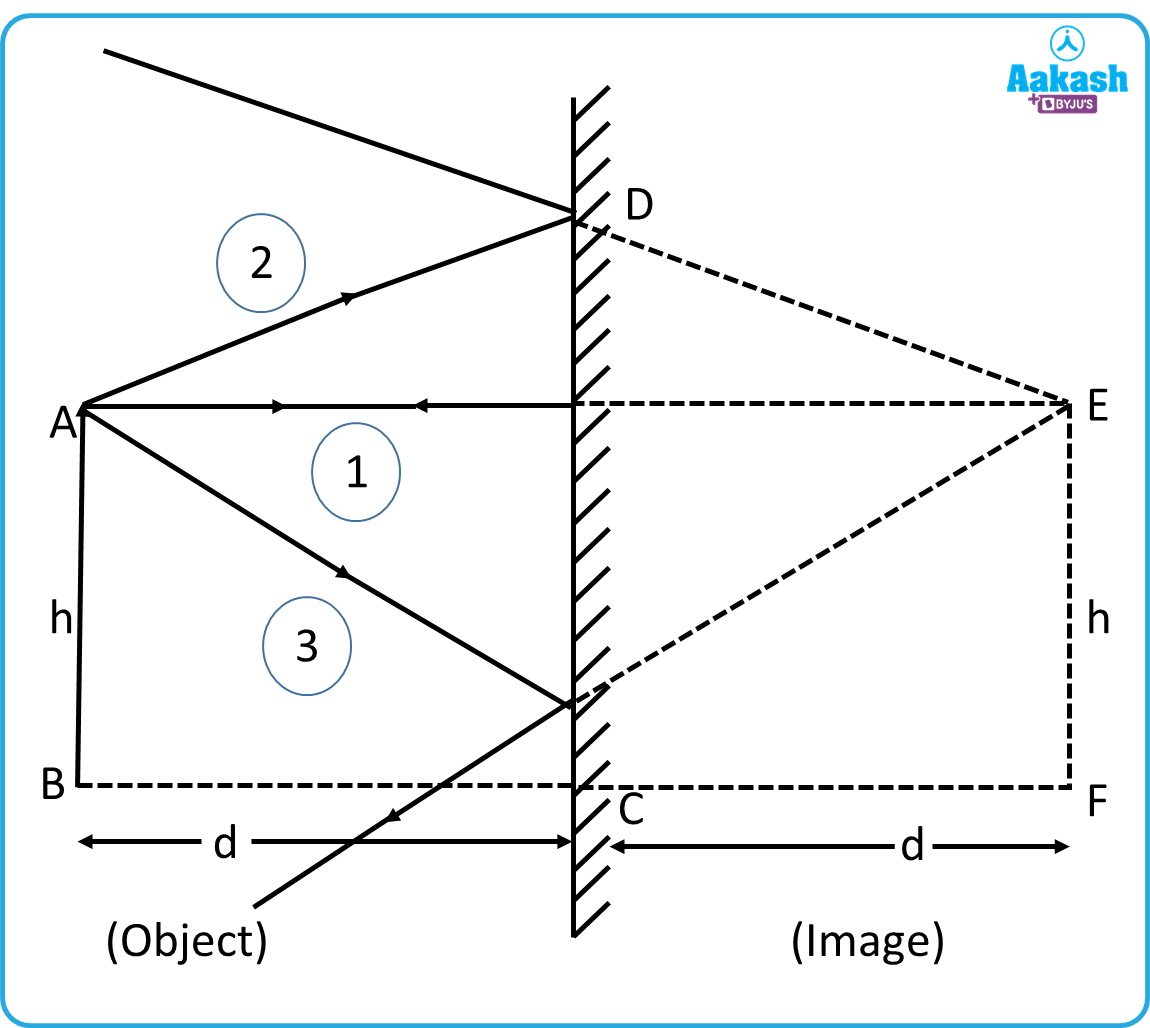Consider a plane mirror CD. An object AB of height h is placed at a distance d in front of the mirror. Focus on the top most point A of the object. Consider three rays incident from that point. Ray 1 incident on the plane perpendicularly, it will reflect back in the same path. The second ray incident on the plane at an angle of incident will reflect at the same angle. Similarly for the third ray incident at some angle will reflect back at the same angle. If we extend any of the two reflected rays inside the mirror, then they will meet at a point E where the image of point A will form.

## Image formed by a plane mirror

To form a complete image of an object first consider any point on the object, say A. If we draw a ray perpendicular to the mirror then after reflection it will return back on the same in the opposite direction. Now draw a ray incident on the mirror at some angle, it will reflect at the angle equal to the angle of incidence. If we extend these reflected rays, they will meet at a point A'.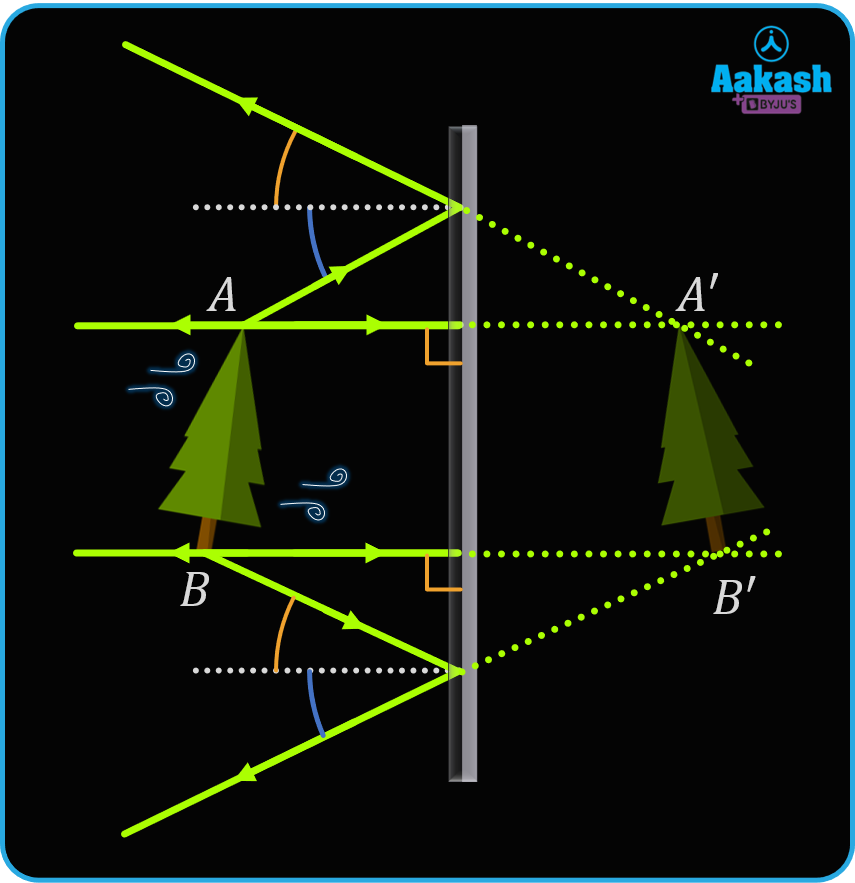Similarly if we draw the ray diagram for all the points on the object and trace the image of all the points then we will find the complete image of an object. The image formed by the plane mirror will be behind the mirror. As all the rays are not actually meeting, but appear to meet, so the final image will be virtual. The distance of the image from the mirror will be equal to the distance of the object from the mirror.

## Characteristics of image formed by plane mirror

From the above discussion, we can conclude that the image formed by a plane mirror has the following characteristics -

1. As the reflected rays appear to meet. So the image will be virtual for a real object.
2. Image formed behind the mirror.
3. The image formed is erect.
4. The size of the image is the same as the object.
5. The image is at the same distance from the mirror as the object.
6. The image is laterally inverted.
7. The image of a moving object will move with the same speed as the object.

## Uses of plane mirror

These are the uses of plane mirror-

1. Plane mirror is used as a dressing mirror.
2. In dental uses, the dentist uses these mirrors for examining the teeth.
3. In solar cooker planes, mirrors are used. The light falling on the plane mirror is completely reflected. This energy is used in cooking the food.
4. kaleidoscopes use plane mirrors with some color which makes a beautiful colored pattern.
5. Periscopes used in submarines which help the submarine driver to navigate underwater.

## Practice problems

Q1. A ray of light incident at an angle of 24o with the surface of a plane mirror. What is the angle of reflection?

A. As shown in figure below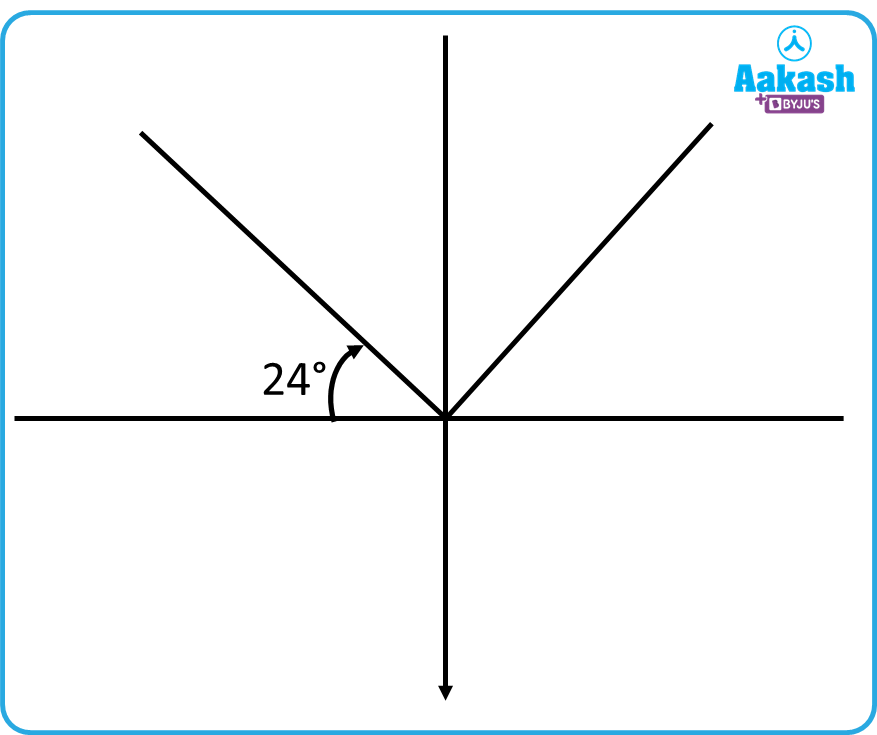Angle of ray with the mirror =24o
Incident angle i = 90- 24= 66o
As we know form the law of reflection i = r
So angle of reflection r = 66o

Q2. A beam of light incident on one of the two plane mirrors kept at right angle as shown in figure. The light beam goes through multiple reflections. If the value of angle A is 36o, then find the value of other angles i.e. B, C and D.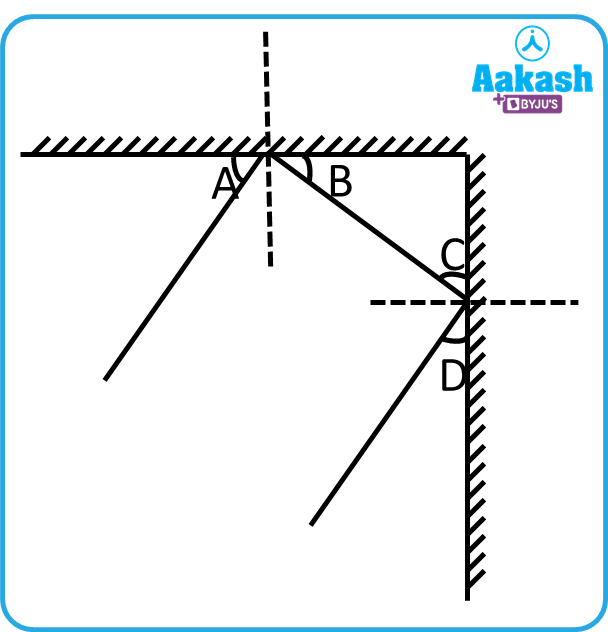A. Given value of A = 36o
From the law of reflection at first mirror i= r1
Subtracting both the side from 90o , We have

A = BH
ence B = 36o
C = 90- B = 90- 36o
C = 54o

Similarly D=54o

Q3. The velocity of the plane mirror is vm = 20 cm s-1. If the mirror is approaching towards you, then find the speed of the image in the mirror.

A. Given,
From the relation of velocities, we have,

Q4. What should be the minimum height of the mirror if a person of 5 ft wants to see the full image of himself?

A. As we know the minimum height of the mirror to see the full image of the object is half of the height of the object.

So, height of the mirror ${\mathbit{H}}_{\mathbit{m}}=\frac{\mathbit{H}}{2}$

Hence, the height of the mirror is 2.5 ft.

## FAQs

Q1. What is the radius of a plane mirror?

A. As we know in the case of a spherical mirror, if an object is placed at infinity then the image will form at focus. If we place an object in front of the mirror at infinity, then the image will also be made at infinity . So the focal length of the plane mirror is infinity.

Q2. Ravi stands in front of a plane mirror at a distance of 2 ft from the mirror. What is the distance of the image from ravi?

A. As Ravi stands at a distance 2 ft from the mirror, so the image will be formed at a distance of 2 ft from the mirror.

Hence the total distance of image from ravi = 2 + 2 ft = 4 ft.

Q3. What is the magnification of the plane mirror?

A. As the plane mirror forms the image of the same size as the object, so the magnification is 1.

Q4. How are plane mirrors made of?

A. Plane mirrors are made of thin glass with one of the sides covered with a reflecting material.Talk to our expert
Resend OTP Timer =
By submitting up, I agree to receive all the Whatsapp communication on my registered number and Aakash terms and conditions and privacy policy